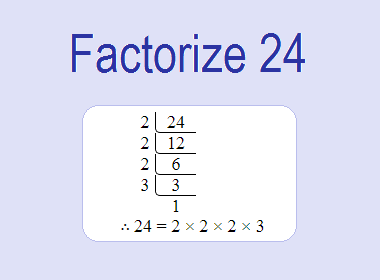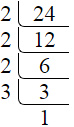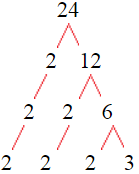# Factorize 24To factorize 24, we need to find the factors of 24. The factors of 24 are 1, 2, 3, 4, 6, 8, 12, and 24 i.e. F24 = {1, 2, 3, 4, 6, 8, 12, 24}. The factors of 24 are those numbers that can divide 24 evenly.

We can check if these numbers are factors of 24 by dividing 24 by each of them. If the result is a whole number, then the number is a factor of 24. Let's do this for each of the numbers listed above:

·        1 is a factor of 24 because 24 divided by 1 is 24.

·        2 is a factor of 24 because 24 divided by 2 is 12.

·        3 is a factor of 24 because 24 divided by 3 is 8.

·        4 is a factor of 24 because 24 divided by 4 is 6.

·        6 is a factor of 24 because 24 divided by 6 is 4.

·        8 is a factor of 24 because 24 divided by 8 is 3.

·        12 is a factor of 24 because 24 divided by 12 is 2.

·        24 is a factor of 24 because 24 divided by 24 is 1.

Therefore, the factors of 24 are 1, 2, 3, 4, 6, 8, 12, and 24.

i.e. F24 = {1, 2, 3, 4, 6, 8, 12, 24}********************

********************

## Prime Factorization of 24

Prime factorization is one of the applications of factors. Prime factorization is the process of finding the prime numbers that can multiply together to give the original number. To find the prime factorization of 24, we can start by dividing it by its smallest prime factor, which is 2. This gives us:

24 ÷ 2 = 12

So, 2 is a factor of 24, and 12 is a factor of 24. Next, we divide 12 by its smallest prime factor, which is again 2. This gives us:

12 ÷ 2 = 6

So, 2 is a factor of 24, and 6 is a factor of 24. Next, we divide 6 by its smallest prime factor, which is again 2. This gives us:

6 ÷ 2 = 3

So, 2 is a factor of 24, and 3 is a factor of 24. Now, we have found all the prime factors of 24, which are 2 and 3. We can write the prime factorization of 24 as:

24 = 2 × 2 × 2 × 3

Or, we can obtain the prime factors of 24 by the following procedure of division as given below:24 = 2 × 2 × 2 × 3

Or, we can obtain the prime factors of 24 by the Factor Tree method as given below:24 = 2 × 2 × 2 × 3

## Factorization of 24

In the above factorization, we have expressed 24 as the product of the prime factors 2 and 3. We can also write this factorization in exponential notation as:

24 = 23 × 3

This notation shows that 2 is a factor of 24, raised to the third power, and 3 is also a factor of 24.

It's important to note that the factorization of a number is not unique. For example, we could also write:

24 = 2 × 2 × 6

In this factorization, we have expressed 24 as the product of factors 2, 2, and 6.

In conclusion, the factors of 24 are 1, 2, 3, 4, 6, 8, 12, and 24, and we can use these factors to factorize 24 into the product of two or more of its factors. One possible factorization of 24 is 2 × 2 × 2 × 3 or 23 × 3. Factorization is a useful tool in many applications of mathematics and is an important concept to understand.